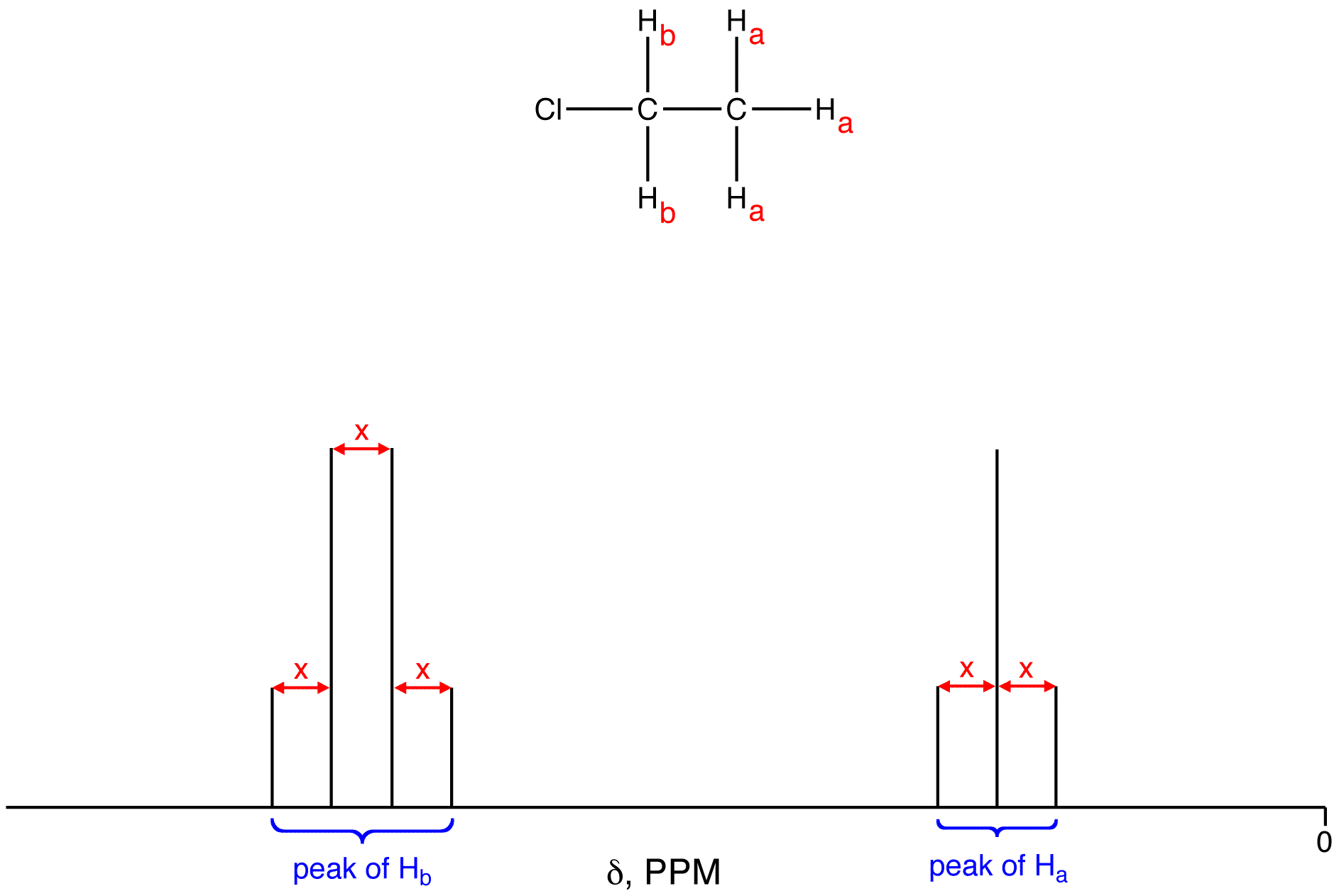# Coupling Constant

•• Contributed by Gamini Gunawardena
• Associate Professor (Chemistry) at Utah Valley University

The distance between any two adjacent lines in the NMR peaks of two sets of equivalent hydrogen nuclei coupled only to each other is the same, which, when expressed in hertz, is called the coupling constant (symbol: J) of the two sets of equivalent hydrogen nuclei.
eg:To convert x, which is in ppm, into the coupling constant, multiply x by the operating frequency, expressed in MHz, of the NMR spectrometer used for the experiment. The x for two sets of equivalent hydrogen nuclei coupled to each other depends on the operating frequency of the spectrometer, but J does not